Custom SearchINFINITESIMALS In chapter 3, we found the slope of a curve at a given point by taking very small increments of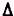y andx, and the slope was said to be equal to. This section will be a continuation of this concept. DEFINITION A variable that approaches 0 as a limit is called an infinitesimal. This may be written as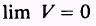or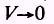and means, as recalled from a previous section of this chapter, that the numerical value of V becomes and remains less than any positive number. If the lim V = L then lim V-L=0 which indicates the difference between a variable and its limit is an infinitesimal. Conversely, if the difference between a variable and a constant is an infinitesimal, then the variable approaches the constant as a limit. EXAMPLE: As x becomes increasingly large, is the term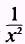an infinitesimal? SOLUTION: By the definition of infinitesimal, ifapproaches 0 as x increases in value, thenis an infinitesimal. We see that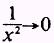and is therefore an infinitesimal. EXAMPLE: As x approaches 2, is the expression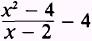an infinitesimal? SOLUTION: By the converse of the definition of infinitesimal, if the difference between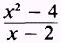and 4 approaches 0, as x approaches 2, the expression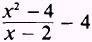is an infinitesimal. By direct substitution we find an indeterminate form; therefore, we make use of our knowledge of indeterminates and write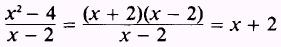and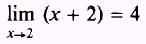The difference between 4 and 4 is 0, so the expression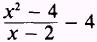is an infinitesimal as x approaches 2. SUMS An infinitesimal is a variable that approaches 0 as a limit. We state thatand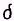, in figure 4-2, are infinitesimals because they both approach 0 as shown. Theorem 1. The algebraic sum of any number of infinitesimals is an infinitesimal. In figure 4-2, asandapproach 0, notice that their sum approaches 0; by definition this sum is an infinitesimal. This approach may be used for the sum of any number of infinitesimals.Figure 4-2.-Sums of infinitesimals. PRODUCTS Theorem 2. The product of any number of infinitesimals is an infinitesimal. In figure 4-3, the product of two infinitesimals,and, is an infinitesimal as shown. The product of any number of infinitesimals is also an infinitesimal by the same approach as shown for two numbers. Theorem 3. The product of a constant and an infinitesimal is an infinitesimal. This may be shown, in figure 4-3, by holding either: orconstant and noticing their product as the variable approaches 0.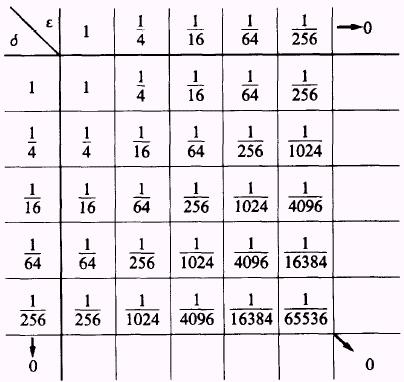Figure 4-3.-Products of infinitesimals.   CONCLUSIONS The term infinitesimal was used to describe the term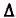x as it approaches zero. The quantityx was called an increment of x, where an increment was used to imply that we made a change in x. Thus x +x indicates that we are holding x constant and changing x by a variable amount which we will call Ax. A very small increment is sometimes called a differential. A smallx is indicated by dx. The differential ofis dand that of y is dy. The limit ofx as it approaches zero is, of course, zero; but that does not mean the ratio of two infinitesimals cannot be a real number or a real function of x. For instance, no matter how smallx is chosen, the ratio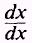will still be equal to 1. In the section on indeterminate forms, a method for evaluating the form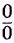was shown. This form results whenever the limit takes the form of one infinitesimal over another. In every case the limit was a real number.Integrated Publishing, Inc. - A (SDVOSB) Service Disabled Veteran Owned Small Business